# Differential Pairs in PCB Transmission Lines: Differential Impedance, Even or Common Mode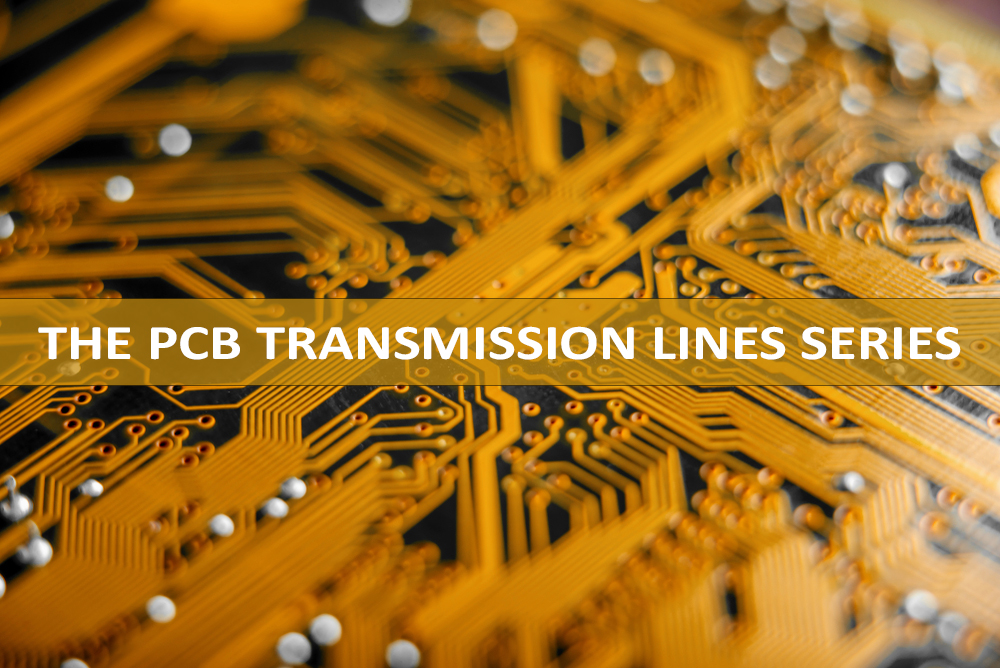This article follows our explanation of differential and common mode signals in differential pairs in PCB transmission lines.

## We will cover:

• Differential impedance
• Even or common mode

### Differential Impedance

The differential impedance is the impedance seen by a purely differential (ie. odd mode) signal over a differential pair. As: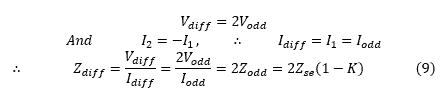Thus, the differential impedance is twice the odd mode impedance. Or the odd mode impedance is half of the differential impedance.

Most often, the only specified requirement of a differential pair is its differential impedance. Typical values for most common differential signal types are 90 ohms differential, 100 ohms differential or 120 ohms differential. In some cases, we can also use 75-ohm differential impedance.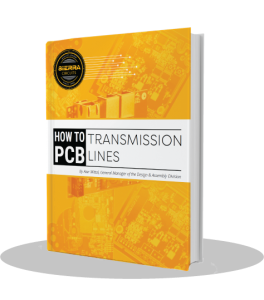### Even or Common Mode

Let’s now take the case when both the lines of the differential pair are excited by a common signal: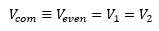Since ‘V1 – V2 = 0’, there is no differential mode signal. As ‘V1 = V2’, ‘I1 = I2’ and the equations (3) and (4) become: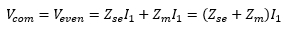Therefore, we can define the even mode impedance ‘Zeven’ as the ratio of the even mode voltage to the even mode current in each line: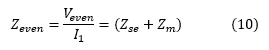As: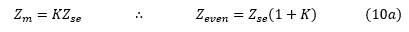And the common mode signal current in the differential pair is ‘I1 + I2 = 2I1’ so that the common mode impedance of the differential pair is: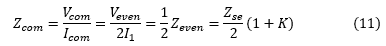Thus, the common mode impedance of the differential pair is half of the even mode impedance of one line. It basically equals two even mode impedances in parallel.

From the equation (10), it is clear that ‘Zeven’ is greater than ‘Zse’. Greater the coupling between the two lines of the pair is, larger will ‘Zeven’ be compared to ‘Zse’.

### Recap: Odd and Even Mode Impedances

Odd and even mode impedances of a differential pair lines are fundamental characteristics of the pair. We can evaluate all other impedances from their values, as illustrated below:

Since: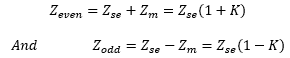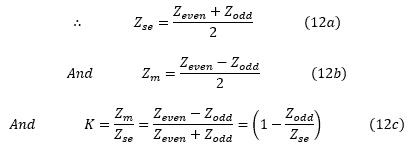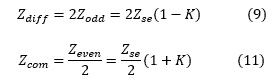In our next article, we will dive a little deeper into the physical parameters of a differential pair.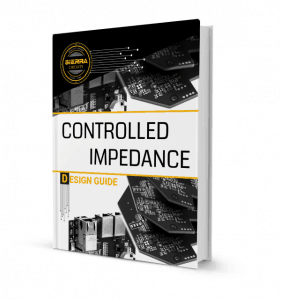Tags: ,

# Differential Pairs in PCB Transmission Lines: Differential Impedance, Even or Common Mode

1.perry says:

Hi, I’d like to design re-driver Circuit. Do I need to consider PCB in my circuit or not. If the answer is yes, how do I get the model for simulation. Thank you!!

2.Don Robison says:

This is great work. I have a question. What does the single ended impedance mean? I physically understand the relevance of even and odd modes since it has to do with the signals on the legs. What does the single-ended impedance mean in terms of signals on legs? Is it the impedance measured on one leg of a diff pair?

1.Zachariah Peterson says:

Don,
The single-ended impedance of a transmission line is the impedance of the line when it is isolated from all other conductors. Think of an infinitely large PCB substrate in vacuum with a single transmission line on it. If you measure the impedance of the line, you are measuring the single-ended impedance.